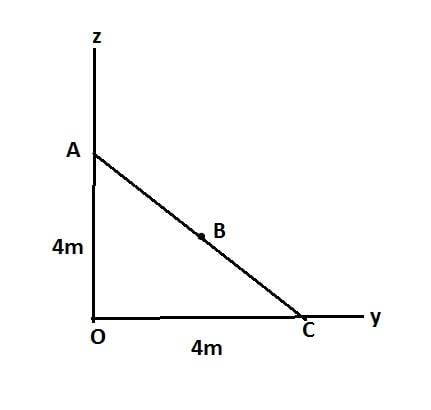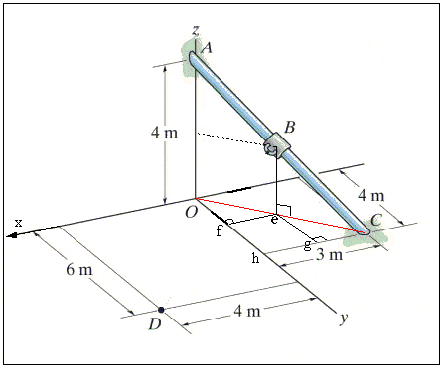# Force acting along a line

sevag00

## Homework Statement

Determine the components of F that act along rod AC and perpendicular to it.
Given: B is located 3 m along the rod from end C.

The problem is that the coordinates of B aren't given so i'm having some trouble in finding the vector components of F along BD. If someone can give me a hint in finding the coordinates of B.

#### Attachments

•Capture.JPG
12.4 KB · Views: 644

## Answers and Replies

Homework Helper
Hint: The coordinates of A and C are given.

sevag00
Still no clue. Is this related to Pythagoras theorem?

Mentor
Still no clue. Is this related to Pythagoras theorem?

Sure, that'll work. Drop a perpendicular from B to the xy plane. Also draw line OC if it helps you to visualize the triangles.

Mentor
Still no clue. Is this related to Pythagoras theorem?
The only thing you don't know is the z coordinate of point B. But you do know that a vector drawn from point D to point B will be perpendicular to a vector drawn from point A to point C. So you know that the cross product of these two vectors must be zero.

Chet

Homework Helper
=sevag00;4545771The problem is that the coordinates of B aren't given...
But you know the coordinates of A and C.

Still no clue. Is this related to Pythagoras theorem?
The rod stretches from A to C.

What are the coordinates of A? What are the coordinates of C?
What is the equation of the line representing the rod?

You are also told:
B is located 3 m along the rod from end C.
What are the coordinates of the point 3m along the rod from C?

sevag00
A(0,0,4) C(-3,4,0)
rAC=(-3-0)i+(4-0)j+(0-4)k

Homework Helper
Do you not know how to find the coordinates of a point 3m back along that line?
How far along the line is that?

Compare with the length of the line: |rAC|=?

Perhaps you'll find it easier to think of in terms of vectors... the vector equation of the line starting at A and going along the beam is:

##\vec{r}_{beam} = \vec{r}\!_A + \lambda\vec{r}\!_{AC}##

i.e. ##(x,y,z) = (0,0,4)+\lambda (-3,4,-4)##

... notice that as λ goes from 0 to 1, rbeam=(x,y,z) goes from point A to point C.

... what does λ have to be to get you the point 3m back from C?

sevag00
Sorry but the equation you just wrote is out of the scope of the chapter.
What does lambda stand for in that equation. Also isn't rbeam=rAC?

Homework Helper
Oh - you don't know about vector equations ... drat!

##\vec{r}_{beam}## is a vector pointing from the origin to a point on the beam.
##\vec{r}\!_{AC}## is a vector pointing along the beam. ##|\vec{r}\!_{AC}|=|AC|##

lambda is a parameter ... ##0<\lambda<1##
when ##\lambda=0## the equation just gives ##(x,y,z)=(0,0,4)## ... which is point A.
when ##\lambda=1## the equation just gives ##(x,y,z)=(-3,4,-4)## ... which is point C.
when ##\lambda## is in-between, then ##(x,y,z)## is a point on the beam in between A and C.

It will get you your answer very directly.

If you use pythagoras and maybe some trig - then you want to construct the triangle OAC - sketch it out. (point O being the origin.)

Point B is 3m up the hypotenuse... you should be able to find it's z-coordinate from that using similar triangles.

You get the x-y coordinates from where B projects onto the OC side.

You'll see what happens if you sketch the side and overhead views of the beam, and mark out where B is.

There's some geometry tricks to notice:
See on the diagram where the 3m sight-line touches the y-axis?
Call that point E.
Then triangle OCE is a 3-4-5 triangle.... so the distance |OC| must be...

So the hypotenuse of OAC must be equal to...

Aside:
You know you could always draw a scale diagram and measure?

Last edited:
sevag00
Let's do it one step at a time. Here is the triangle.I'm not sure if B is the midpoint of AC. What do you say?
Also, I didn't get how the z coordinate of B is 3.
Also, AC=5.65

Mentor
Harken back to your geometry classes; invoke similar triangles:

https://www.physicsforums.com/attachment.php?attachmentid=63213&stc=1&d=1382439539
$$\frac{Be}{BC} = \frac{AO}{AC}$$
The other coordinates of B can be found by recognizing other similar triangle opportunities in the setup.

sevag00
Where is point E on the triangle?

Mentor
Where is point E on the triangle?

I added point e to your diagram when I dropped the vertical line from B to the xy plane (where the base of the triangle sits).

sevag00
Sorry, i can't view that image. The attachment is broken.

Mentor
Sorry, i can't view that image. The attachment is broken.

Really? It shows up fine for me in post #12. The link looks fine.

In words then: Take your drawing and drop a vertical line from B to the base of the triangle. Call the point where it meets the base point e. BeC then forms a triangle similar to AOC. Use ratios to find the length of Be.

sevag00
zB 2 is being negative when i subtract.

Mentor
I'm not sure what it is you're trying to find with these calculations. I thought you were going to find the coordinates of the point B.

Even so, it looks to me like the value you're plugging in for the length AC is not correct. How did you obtain it?

sevag00
Using Pythagoras theorem i calculated the length of AC which is 5.65.
I made a mistake early on, it should be;
BC = (-3-x)i + (4-2.12)j + (0-2.12)k
By the above equation im trying to get the x coordinate of B.

Last edited:
Mentor
Well let's see. The coordinates of the endpoints of AC are: A = (0,0,4) ; C = (-3, 4, 0). Then

$$|AC| = \sqrt{(0 - (-3))^2 + (0 - 4)^2 + (4 - 0)^2} = \sqrt{41} \approx 6.403$$

sevag00
Aha. Then Be = eC = 1.874. Which are also the y and z components of B
Using my method above we plug in 1.874 instead of 2.12.
We get the x coordinate of B, right?

Mentor
Why do you say that Be = eC? Are you claiming that AC makes a 45° angle with the xy-plane?

Here's the original diagram again with the force F removed for clarity, and additional annotations for easy reference:The y-coordinate of B will be determined by the length of the line segment Of. the x-coordinate by the length of the line segment fe (and according to the directions of the axes, you want -fe).

#### Attachments

sevag00
Are we going to use similar triangles again?

Mentor
Are we going to use similar triangles again?

Well, you get to choose the method. I've suggested using similar triangles, and Simon Bridge has suggested a vector approach. Either will get the job done, although Simon's is speedier and you will still need to use vectors to finish the problem.

sevag00
OC = 5, I have Be and BC, i get eC. Then OC - eC = Oe
$$\frac{Oe}{Of} = \frac{AC}{AO}$$
We get Of. Then Pythagoras on triangle Ofe, we get fe.
Is this approach correct?

Mentor
I wouldn't trust that angle OAC is the same as angle fOe without proof.

Instead I would look to guaranteed similar triangles Ofe and OhC. You know length OC (5), and you can find Oe easily enough from the way AC is divided into parts by point B.

sevag00
Is this what you're saying?
$$\frac{Oe}{Of} = \frac{OC}{OH}$$

Mentor
That would work just fine.

sevag00
Ok. Thanks for the help.

sevag00
But i don't know if my professor knows that the students know or remember similar triangles. I'm curious to know how is he going to solve this problem during class.

Mentor
But i don't know if my professor knows that the students know or remember similar triangles. I'm curious to know how is he going to solve this problem during class.

Everyone is expected to remember their geometryI would bet he'll use vectors. To complete the problem without vectors would be very taxing.

Finding the location of point B using geometry is pretty straightforward. When the force F is added in and you start looking for components parallel to and perpendicular to the pipe, the geometry soon becomes tedious (although still doable, the amount of effort is just begging for errors to slip in). Vectors will take care of it all in just a few lines!